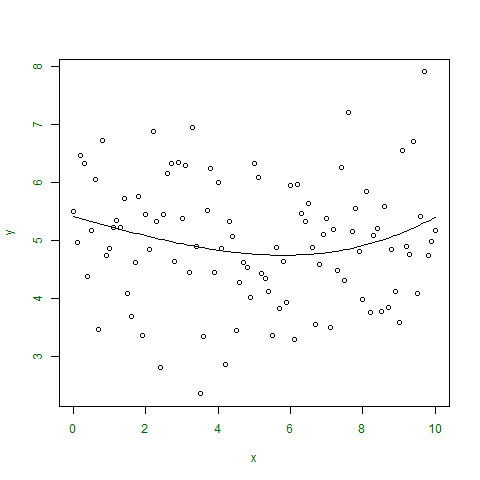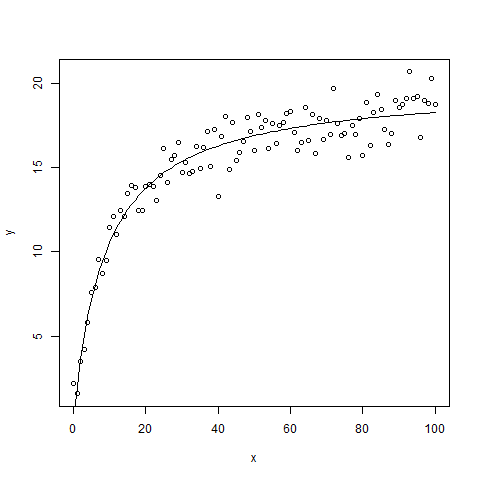# R – NonLinear Least Square

In non-linear function, the points plotted on the graph are not linear and thus, do not give a curve or line on the graph. So, non-linear regression analysis is used to alter the parameters of the function to obtain a curve or regression line that is closed to your data.
To perform this, Non-Linear Least Square approach is used to minimize the total sum of squares of residual values or error values i.e., the difference between vertical points on the graph from regression line and will fit the non-linear function accordingly.

Mathematical Formula:where,

r is residual or error value between 2 points.

Above mathematical function to find minimum residual function can be performed in R using resid() function.

Regression analysis is widely used in all types of business issues to perform a smart decision or predicting the future by altering a factor of their business.
In R language, Non-linear Least Square function is represented as –
Syntax:

nls(formula, start)


where,

formula indicates the model formula i.e., non-linear function
start is a list of starting estimates

Note: To know about more optional parameters of nls(), use below command in R console –

help("nls")


Example 1 :
In this example, a non-linear function is taken and plotted on the graph as points.

 # defining x and y coordinates  x <- seq(0, 10, 0.1)  y <- rnorm(101, 5, 1)      # output to be present as PNG file  png(file ="nls.png")      # Taking the model to get fitted  m <- nls(y~a * x ^ 3 + b * x + c,            start = list(a = 1, b = 2, c = 1))      # plot the graph  plot(x, y, col.lab ="darkgreen",              col.axis ="darkgreen")      # plot the graph with new fitting line  # or regression line  lines(x, predict(m))      # saving the file  dev.off()      # print minimum residual or error value  print(sum(resid(m)^2))

Output :

 106.4507Example 2 :
In this example, below code is accepting a non-linear function as shown:Further plotting the points and regression line and also, finding out the goodness of fit also by using cor() method.

 # creating sequence of 101 values from 0 to 100  x <- seq(0, 100, 1)      y<-((runif(1, 10, 20)*x)/(runif(1, 0, 10) + x)) +                            rnorm(101, 0, 1)      # output to be present as PNG file  png(file ="nls2.png")      # using starting values in nls() function  # to not get a warning  m<-nls(y~a * x/(b + x), start = list(a = 1, b = 2))      # goodness of fit  cor(y, predict(m))      # minimized residual value  sum(resid(m)^2)      # plotting points on graph  plot(x, y)      # finding regression line  lines(x, predict(m))      # saving the file  dev.off()

Output :

 0.9622681
 108.1481My Personal Notes arrow_drop_upBlockchain Enthusiast

If you like GeeksforGeeks and would like to contribute, you can also write an article using contribute.geeksforgeeks.org or mail your article to contribute@geeksforgeeks.org. See your article appearing on the GeeksforGeeks main page and help other Geeks.

Please Improve this article if you find anything incorrect by clicking on the "Improve Article" button below.

Article Tags :

1

Please write to us at contribute@geeksforgeeks.org to report any issue with the above content.